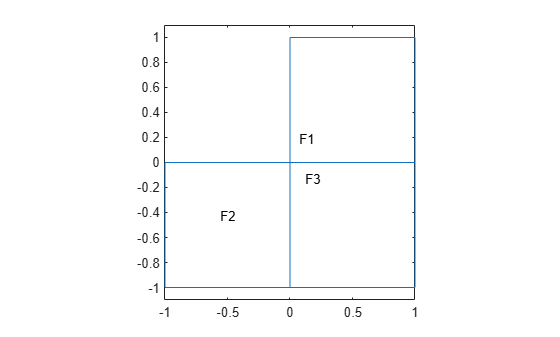# findCoefficients

Locate active PDE coefficients

## Syntax

``CA = findCoefficients(coeffs,RegionType,RegionID)``

## Description

example

````CA = findCoefficients(coeffs,RegionType,RegionID)` returns the active coefficient assignment `CA` for the coefficients in the specified region.```

## Examples

collapse all

Create a PDE model that has a few subdomains.

```model = createpde(); geometryFromEdges(model,@lshapeg); pdegplot(model,'FaceLabels','on') ylim([-1.1,1.1]) axis equal```Set coefficients on each pair of regions.

```specifyCoefficients(model,'m',0,'d',0,'c',12,'a',0,'f',1,'Face',[1,2]); specifyCoefficients(model,'m',0,'d',0,'c',13,'a',0,'f',2,'Face',[1,3]); specifyCoefficients(model,'m',0,'d',0,'c',23,'a',0,'f',3,'Face',[2,3]);```

Check the coefficient specification for region 1.

```coeffs = model.EquationCoefficients; ca = findCoefficients(coeffs,'Face',1)```
```ca = CoefficientAssignment with properties: RegionType: 'face' RegionID: [1 3] m: 0 d: 0 c: 13 a: 0 f: 2 ```

## Input Arguments

collapse all

Model coefficients, specified as the `EquationCoefficients` property of a PDE model. Coefficients can be complex numbers.

Example: `model.EquationCoefficients`

Geometric region type, specified as `'Face'` for a 2-D model, or `'Cell'` for a 3-D model.

Example: ```ca = findCoefficients(coeffs,'Face',[1,3])```

Data Types: `char` | `string`

Region ID, specified as a vector of positive integers. View the subdomain labels for a 2-D model using `pdegplot(model,'FaceLabels','on')`. Currently, there are no subdomains for 3-D models, so the only acceptable value for a 3-D model is `1`.

Example: ```ca = findCoefficients(coeffs,'Face',[1,3])```

Data Types: `double`

## Output Arguments

collapse all

Coefficient assignment, returned as a CoefficientAssignment Properties object.

## Version History

Introduced in R2016a